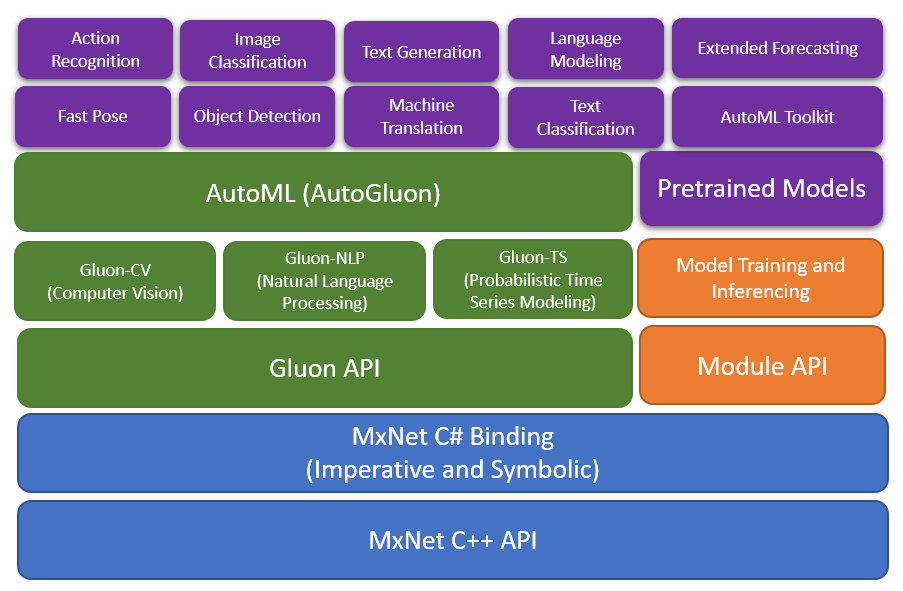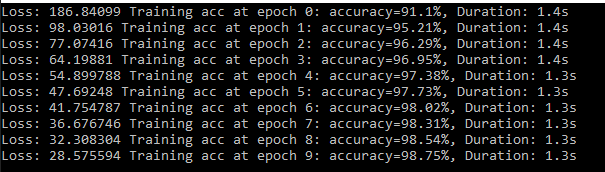# Work In Progress version 2.0.

There are many breaking change as per RFC: https://github.com/apache/incubator-mxnet/issues/16167. With this change we are introducing NumPy-compatible coding experience into MXNet

# Apache MXNet (incubating) for Deep Learning

Apache MXNet (incubating) is a deep learning framework designed for both efficiency and flexibility. It allows you to mix symbolic and imperative programming to maximize efficiency and productivity. At its core, MXNet contains a dynamic dependency scheduler that automatically parallelizes both symbolic and imperative operations on the fly. A graph optimization layer on top of that makes symbolic execution fast and memory efficient. MXNet is portable and lightweight, scaling effectively to multiple GPUs and multiple machines.

MxNet.Sharp

MxNet.Sharp is a CSharp binding coving all the Imperative, Symbolic and Gluon API's with an easy to use interface. The Gluon library in Apache MXNet provides a clear, concise, and simple API for deep learning. It makes it easy to prototype, build, and train deep learning models without sacrificing training speed.

High Level Arch## A New NumPy Interface for MxNet#

The MXNet community is pleased to announce a new NumPy interface for MXNet that allows developers to retain the familiar syntax of NumPy, while leveraging performance gains from accelerated computing on GPUs and asynchronous execution on CPUs and GPUs, in addition to automatic differentiation for differentiable NumPy ops through MxNet.Autograd.

The new NumPy interface from MXNet, MxNet.Numpy, is intended to be a drop-in replacement for NumPy, as such mxnet.numpy supports many familiar numpy.ndarray operations necessary for developing machine learning or deep learning models and operations are continually being added.

## Work List

• [x] Project prep work for v2
• [x] Adding numpy ndarray array object and properties
• [x] Implementing numpy creation function
• [x] Implementing numpy elementwise
• [x] Numpy basic indexing
• [ ] Numpy advance indexing
• [x] Nummy linear algebra functions
• [x] Numpy manipulation functions
• [x] Numpy search and sorting functions
• [ ] Numpy statistical functions
• [ ] Gluon updates with numpy ops
• [x] Implement numpy extension functions for neural network
• [ ] Gluon probability
• [ ] Mxnet 2 Onnx and Onnx 2 Mxnet
• [ ] More examples
• [ ] Unit testing
• [x] CI Builds

## MxNet.Numpy Vs NumPy Performance

Lets consider simple test to see the performance difference. I will keep adding more scenarios and with GPU test as well.

### Scenario 1

```using MxNet;
using MxNet.Numpy;
using System;

namespace PerfTest
{
class Program
{
static void Main(string[] args)
{
DateTime start = DateTime.Now;
var x = np.random.uniform(size: new Shape(3000, 3000));
var y = np.random.uniform(size: new Shape(3000, 3000));
var d = np.dot(x, y);
npx.waitall();
Console.WriteLine(d.shape);
Console.WriteLine("Duration: " + (DateTime.Now - start).TotalMilliseconds / 1000);
}
}
}
```
```import numpy as np
import time

start_time = time.time()
x = np.random.uniform(0, 1, (3000, 1000))
y = np.random.uniform(0, 1, (3000, 3000))
d = np.dot(x, y);
#d = 0.5 * np.sqrt(x) + np.sin(y) * np.log(x) - np.exp(y)
print(d.shape)
print("--- %s sec ---" % (time.time() - start_time))
```

### Scenario 2

```using MxNet;
using MxNet.Numpy;
using System;

namespace PerfTest
{
class Program
{
static void Main(string[] args)
{
DateTime start = DateTime.Now;
var x = np.random.uniform(size: new Shape(30000, 10000));
var y = np.random.uniform(size: new Shape(30000, 10000));
var d = 0.5f * np.sqrt(x) + np.sin(y) * np.log(x) - np.exp(y);
npx.waitall();
Console.WriteLine(d.shape);
Console.WriteLine("Duration: " + (DateTime.Now - start).TotalMilliseconds / 1000);
}
}
}
```
```import numpy as np
import time

start_time = time.time()
x = np.random.uniform(0, 1, (30000, 10000))
y = np.random.uniform(0, 1, (30000, 10000))
d = 0.5 * np.sqrt(x) + np.sin(y) * np.log(x) - np.exp(y)
print(d.shape)
print("--- %s sec ---" % (time.time() - start_time))
```
Scenario MxNet CPU NumPy
1 1.2247 145.4460
2 24.4994 14.3616

## Nuget

Install the package: Install-Package MxNet.Sharp

https://www.nuget.org/packages/MxNet.Sharp

Add the MxNet redistributed package available as per below.

Important: Make sure your installed CUDA version matches the CUDA version in the nuget package.

Check your CUDA version with the following command:

``````nvcc --version
``````

You can either upgrade your CUDA install or install the MXNet package that supports your CUDA version.

MxNet Version Build: https://github.com/apache/incubator-mxnet/releases/tag/1.5.0

Win-x64 Packages

Type Name Nuget
MxNet-CPU MxNet CPU Version Install-Package MxNet.Runtime.Redist
MxNet-MKL MxNet CPU with MKL Install-Package MxNet-MKL.Runtime.Redist
MxNet-CU101 MxNet for Cuda 10.1 and CuDnn 7 Install-Package MxNet-CU101.Runtime.Redist
MxNet-CU101MKL MxNet for Cuda 10.1 and CuDnn 7 Install-Package MxNet-CU101MKL.Runtime.Redist
MxNet-CU100 MxNet for Cuda 10 and CuDnn 7 Install-Package MxNet-CU100.Runtime.Redist
MxNet-CU100MKL MxNet with MKL for Cuda 10 and CuDnn 7 Install-Package MxNet-CU100MKL.Runtime.Redist
MxNet-CU92 MxNet for Cuda 9.2 and CuDnn 7 Install-Package MxNet-CU100.Runtime.Redist
MxNet-CU92MKL MxNet with MKL for Cuda 9.2 and CuDnn 7 Install-Package MxNet-CU92MKL.Runtime.Redist
MxNet-CU80 MxNet for Cuda 8.0 and CuDnn 7 Install-Package MxNet-CU100.Runtime.Redist
MxNet-CU80MKL MxNet with MKL for Cuda 8.0 and CuDnn 7 Install-Package MxNet-CU80MKL.Runtime.Redist

Linux-x64 Packages

Type Name Nuget
MxNet-CPU MxNet CPU Version Install-Package MxNet.Linux.Runtime.Redist
MxNet-MKL MxNet CPU with MKL Install-Package MxNet-MKL.Linux.Runtime.Redist
MxNet-CU101 MxNet for Cuda 10.1 and CuDnn 7 Yet to publish
MxNet-CU101MKL MxNet for Cuda 10.1 and CuDnn 7 Yet to publish
MxNet-CU100 MxNet for Cuda 10 and CuDnn 7 Yet to publish
MxNet-CU100MKL MxNet with MKL for Cuda 10 and CuDnn 7 Yet to publish
MxNet-CU92 MxNet for Cuda 9.2 and CuDnn 7 Yet to publish
MxNet-CU92MKL MxNet with MKL for Cuda 9.2 and CuDnn 7 Yet to publish
MxNet-CU80 MxNet for Cuda 8.0 and CuDnn 7 Yet to publish
MxNet-CU80MKL MxNet with MKL for Cuda 8.0 and CuDnn 7 Yet to publish

OSX-x64 Packages

Type Name Nuget
MxNet-CPU MxNet CPU Version Yet to publish
MxNet-MKL MxNet CPU with MKL Yet to publish
MxNet-CU101 MxNet for Cuda 10.1 and CuDnn 7 Yet to publish
MxNet-CU101MKL MxNet for Cuda 10.1 and CuDnn 7 Yet to publish
MxNet-CU100 MxNet for Cuda 10 and CuDnn 7 Yet to publish
MxNet-CU100MKL MxNet with MKL for Cuda 10 and CuDnn 7 Yet to publish
MxNet-CU92 MxNet for Cuda 9.2 and CuDnn 7 Yet to publish
MxNet-CU92MKL MxNet with MKL for Cuda 9.2 and CuDnn 7 Yet to publish
MxNet-CU80 MxNet for Cuda 8.0 and CuDnn 7 Yet to publish
MxNet-CU80MKL MxNet with MKL for Cuda 8.0 and CuDnn 7 Yet to publish

## Gluon MNIST Example

```var mnist = TestUtils.GetMNIST(); //Get the MNIST dataset, it will download if not found
var batch_size = 200; //Set training batch size
var train_data = new NDArrayIter(mnist["train_data"], mnist["train_label"], batch_size, true);
var val_data = new NDArrayIter(mnist["test_data"], mnist["test_label"], batch_size);

// Define simple network with dense layers
var net = new Sequential();

//Set context, multi-gpu supported
var gpus = TestUtils.ListGpus();
var ctx = gpus.Count > 0 ? gpus.Select(x => Context.Gpu(x)).ToArray() : new[] {Context.Cpu(0)};

//Initialize the weights
net.Initialize(new Xavier(magnitude: 2.24f), ctx);

//Create the trainer with all the network parameters and set the optimizer
var trainer = new Trainer(net.CollectParams(), new Adam());

var epoch = 10;
var metric = new Accuracy(); //Use Accuracy as the evaluation metric.
var softmax_cross_entropy_loss = new SoftmaxCELoss();
float lossVal = 0; //For loss calculation
for (var iter = 0; iter < epoch; iter++)
{
var tic = DateTime.Now;
// Reset the train data iterator.
train_data.Reset();
lossVal = 0;

// Loop over the train data iterator.
while (!train_data.End())
{
var batch = train_data.Next();

// Splits train data into multiple slices along batch_axis
// and copy each slice into a context.
var data = Utils.SplitAndLoad(batch.Data, ctx, batch_axis: 0);

// Splits train labels into multiple slices along batch_axis
// and copy each slice into a context.
var label = Utils.SplitAndLoad(batch.Label, ctx, batch_axis: 0);

var outputs = new NDArrayList();

// Inside training scope
{
outputs = Enumerable.Zip(data, label, (x, y) =>
{
var z = net.Call(x);

// Computes softmax cross entropy loss.
NDArray loss = softmax_cross_entropy_loss.Call(z, y);

// Backpropagate the error for one iteration.
loss.Backward();
lossVal += loss.Mean();
return z;
}).ToList();
}

metric.Update(label, outputs.ToArray());

// Make one step of parameter update. Trainer needs to know the
// batch size of data to normalize the gradient by 1/batch_size.
trainer.Step(batch.Data.Shape);
}

var toc = DateTime.Now;

// Gets the evaluation result.
var (name, acc) = metric.Get();

// Reset evaluation result to initial state.
metric.Reset();
Console.Write(\$"Loss: {lossVal} ");
Console.WriteLine(\$"Training acc at epoch {iter}: {name}={(acc * 100).ToString("0.##")}%, Duration: {(toc - tic).TotalSeconds.ToString("0.#")}s");
}
```

Reached accuracy of 98% within 6th epoch.# Documentation (In Progress)

https://mxnet.tech-quantum.com/

Get A Weekly Email With Trending Projects For These Topics
No Spam. Unsubscribe easily at any time.
c-sharp (12,224
deep-learning (3,906
machine-learning (3,567
neural-network (735
object-detection (475
image-classification (176
mxnet (106
gluon (28